# 机器学习：通过按键声音破解你的密码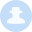iso60001  438天前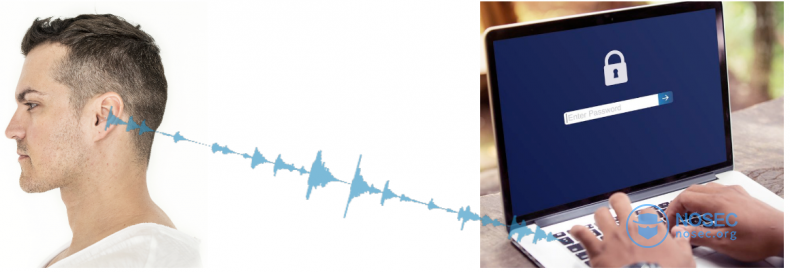## 大纲

1. 数据收集和准备

2. 训练和评估

3. 测试和误差分析（提高模型精度）

4. 总结成果，上传GitHub

## 数据收集## 数据准备Quicktime会将录制的音频保存为mp4格式，那么我们首先将mp4转换为wav格式，因为有现成的Python库可以处理wav文件。上图中的每个峰值对应一个击键，因此可以使用`split_on_silence`函数将音频分割成单独的数据块，这样每个数据块只包含一个字母。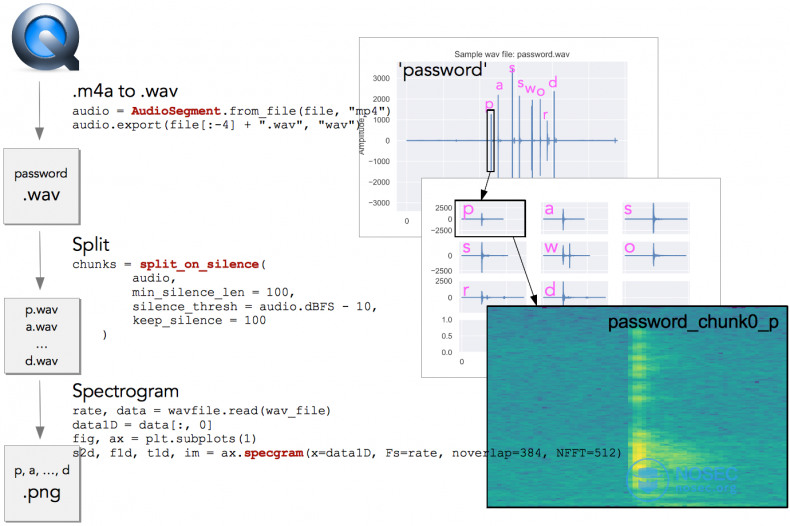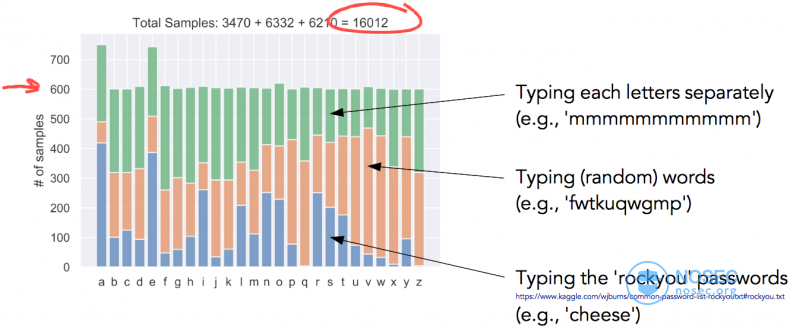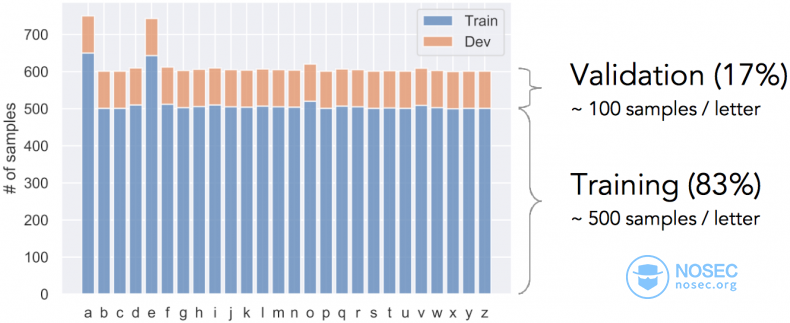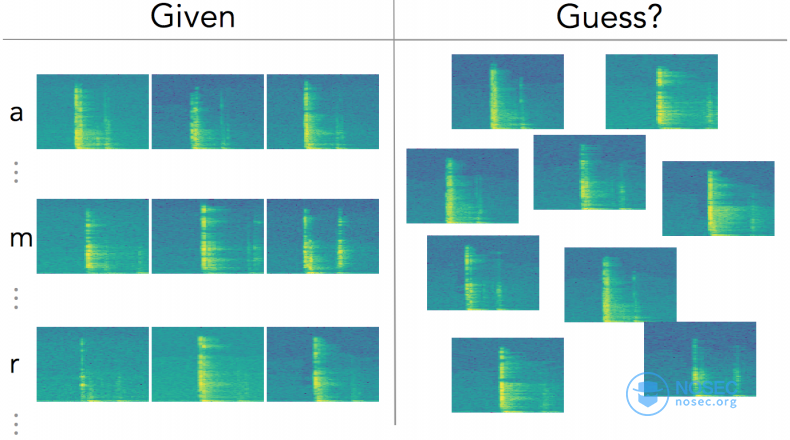## 训练和验证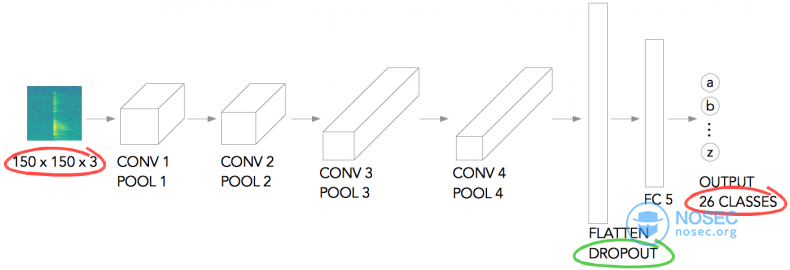``````model = tf.keras.models.Sequential([
# 1st convolution
tf.keras.layers.Conv2D(64, (3,3), activation='relu', input_shape=(150, 150, 3)),
tf.keras.layers.MaxPooling2D(2, 2),

# 2nd convolution
tf.keras.layers.Conv2D(64, (3,3), activation='relu'),
tf.keras.layers.MaxPooling2D(2,2),

# 3rd convolution
tf.keras.layers.Conv2D(128, (3,3), activation='relu'),
tf.keras.layers.MaxPooling2D(2,2),

# 4th convolution
tf.keras.layers.Conv2D(128, (3,3), activation='relu'),
tf.keras.layers.MaxPooling2D(2,2),

# Flatten the results to feed into a DNN
tf.keras.layers.Flatten(),
tf.keras.layers.Dropout(0.5),

# FC layer
tf.keras.layers.Dense(512, activation='relu'),

# Output layer
tf.keras.layers.Dense(26, activation='softmax')
])
``````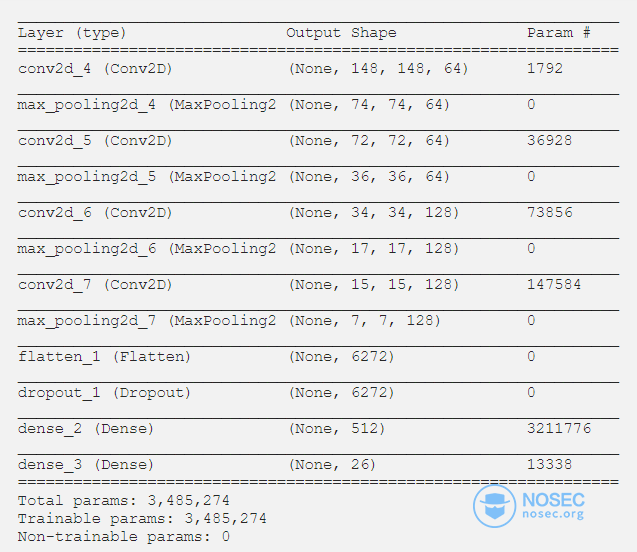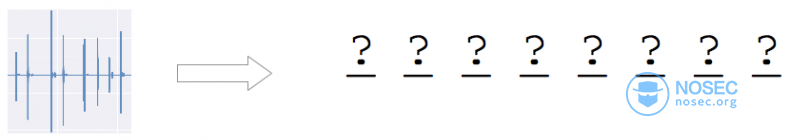## 测试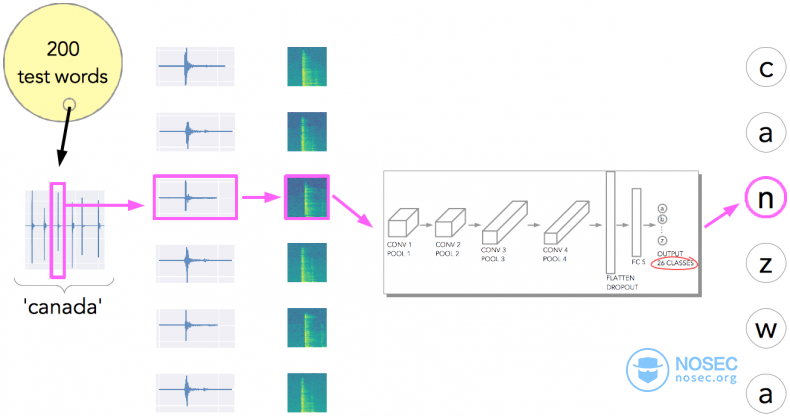## 误差分析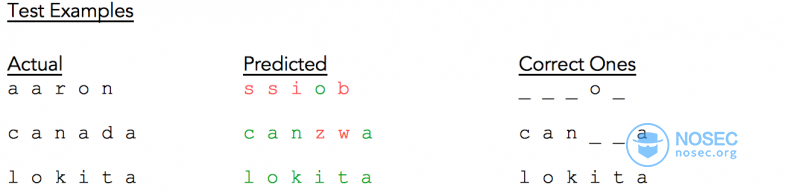`canada`为例，如果使用单词拼写检查函数进行处理，会有改善么？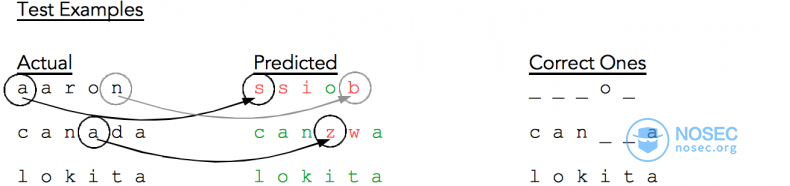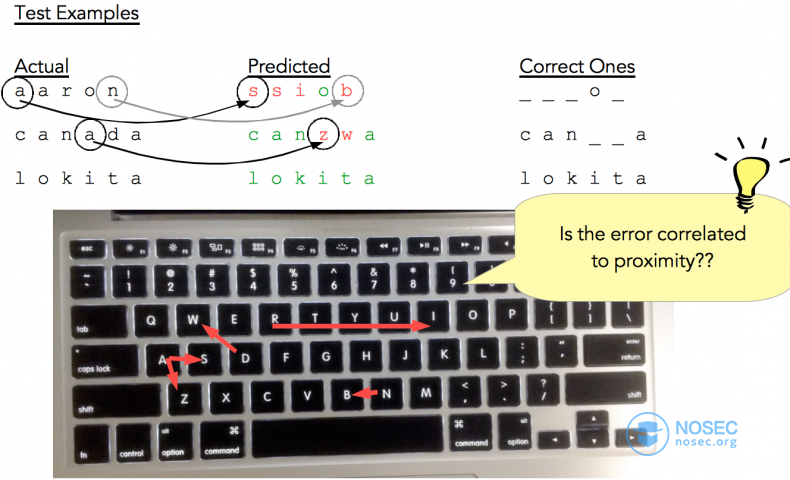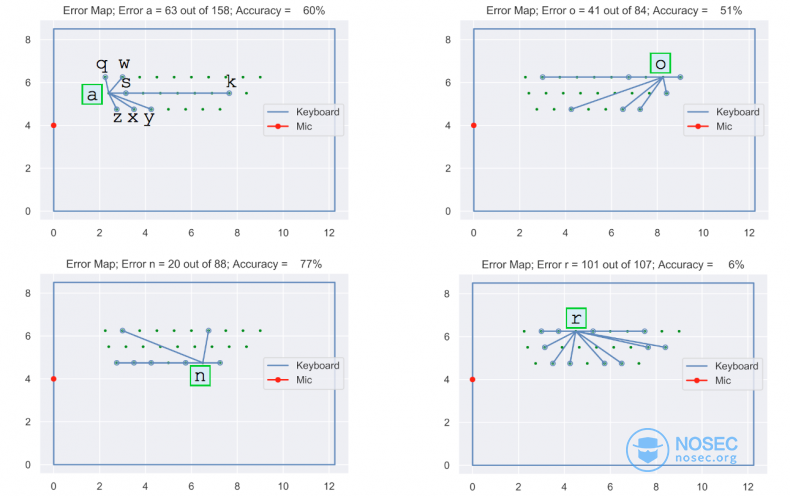`d_ref`为正确字母到麦克风的距离，`d_predicted`为预测字母到麦克风的距离，而`d``d_ref``d_predicted`差的绝对值。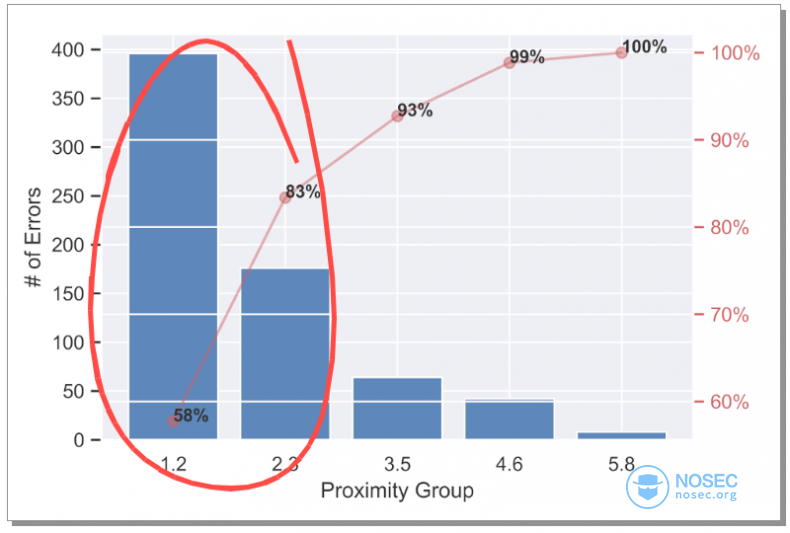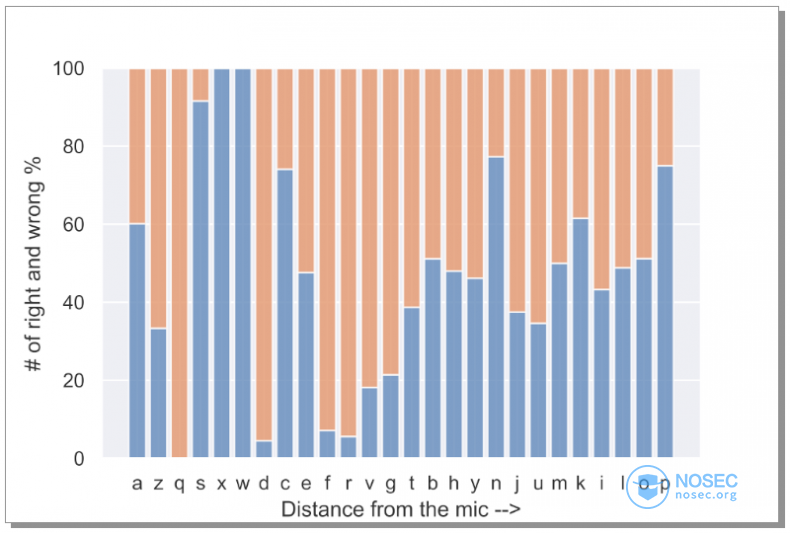## 其它改进

• 打字速度

• 特殊按键的（回车和大小写等）影响

• 背景噪音

• 输入源和麦克风品牌的影响

• 是否可以收集其他音源？（键盘震动？）

## 结论

``````本文由白帽汇整理并翻译，不代表白帽汇任何观点和立场

``````

#### 最新评论